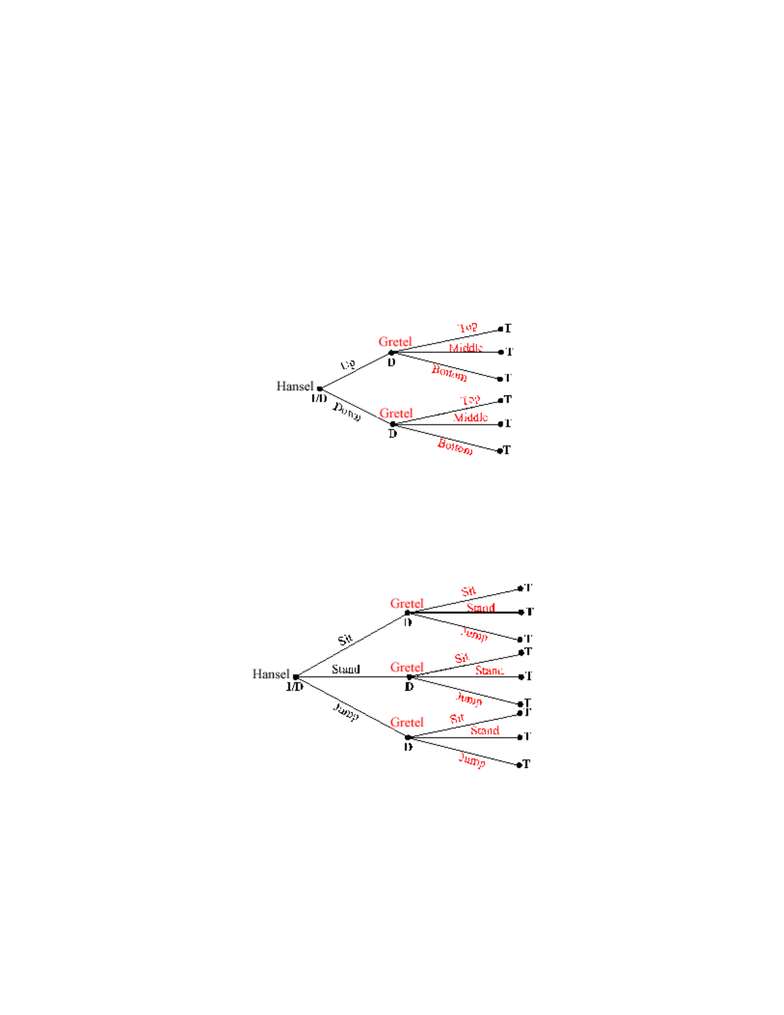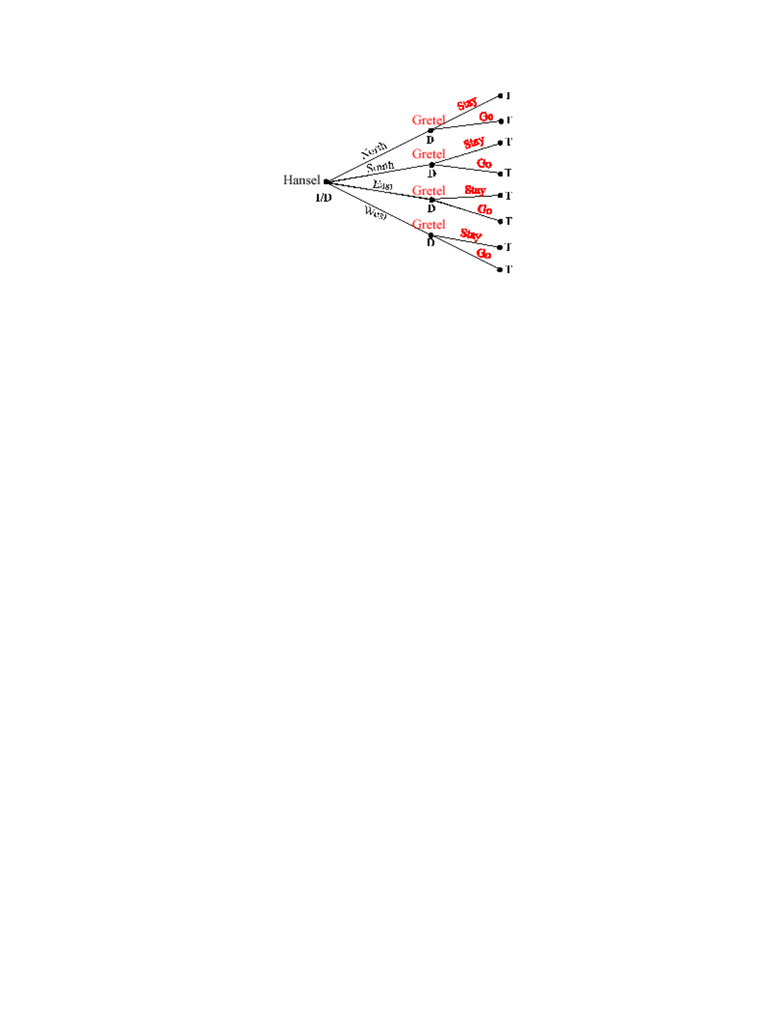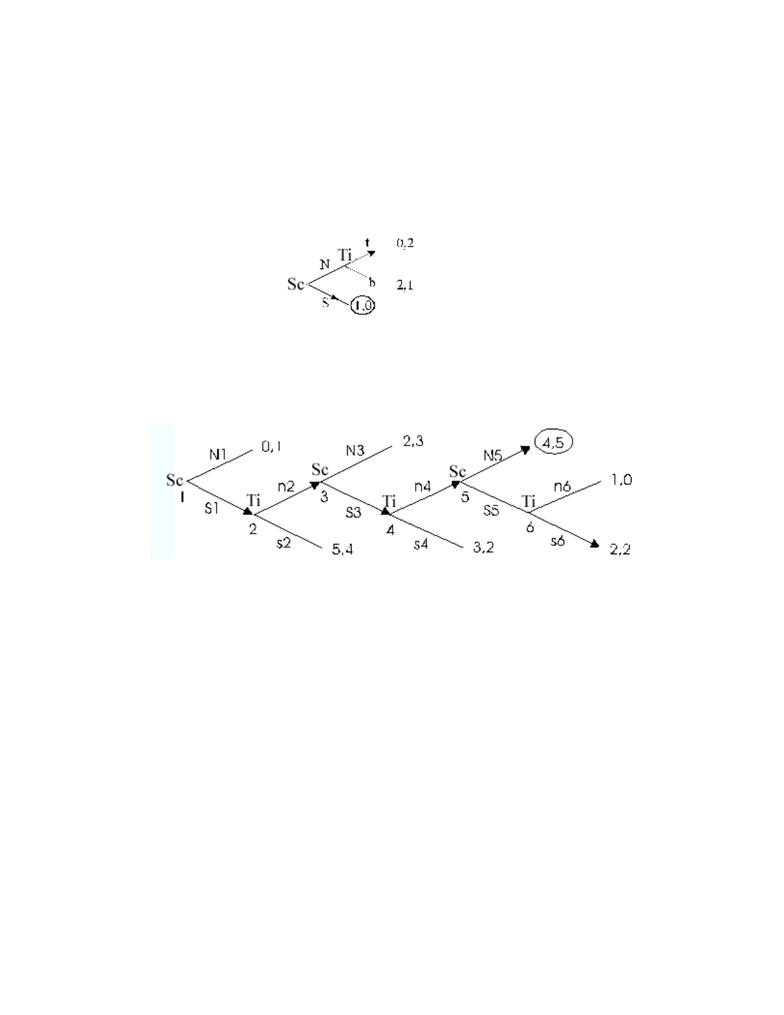# ch03_solutions_solved edit.doc

40 views10 pages
School
Department
CourseSolutions to Chapter 3 Exercises
SOLVED EXERCISES
S1. (a) There is one initial node (I) for Hansel making the first move; three decision nodes (D)
including the initial node, which represent the points where either Hansel or Gretel make a decision; and
six terminal nodes (T).
(b) There is one initial node (I) for Hansel making the first move; four decision nodes (D)
including the initial node, which represent the nodes where Hansel or Gretel make a decision; and nine
terminal nodes (T).
(c) There is one initial node (I) for Hansel making the first move; five decision nodes (D)
including the initial node, which represent the nodes where Hansel or Gretel make a decision; and eight
terminal nodes (T).
Solutions to Chapter 3 Solved Exercises 1 of 10
Unlock document

This preview shows pages 1-3 of the document.
Unlock all 10 pages and 3 million more documents.S2. For this question, remember that actions with the same label, if taken at different nodes, are
different components of a strategy. To clarify the answers, the nodes on the trees are labeled 1, 2, etc. (in
addition to showing the name of the player acting there). Actions in a strategy are designated as N1
(meaning N at node 1), etc. The trees are below in the solutions to Exercise S3. Numbering of nodes
begins at the far left and proceeds to the right, with nodes equidistant to the right of the initial node and
numbered from top to bottom.
(a) Scarecrow has two strategies: (1) N or (2) S. Tinman has two strategies: (1) t if
Scarecrow plays N, or (2) b if Scarecrow plays N.
(b) Scarecrow has two actions at three different nodes, so Scarecrow has eight strategies,
2•2•2=8. To describe the strategies accurately, we must specify a player’s action at each decision node.
Scarecrow decides at nodes 1, 3, and 5, so we will label a strategy by listing the action and the node
number. For example, to describe Scarecrow choosing N at each node, we write (N1, N3, N5).
Accordingly, the eight strategies for Scarecrow are: (N1, N3, N5), (N1, N3, S5), (N1, S3, N5), (S1, N3,
N5), (N1, S3, S5), (S1, N3, S5), (S1, S3, N5), and (S1, S3, S5).
Tinman has two actions at three different nodes, so Tinman also has eight strategies, 2•2•2=8.
Tinman’s strategies are: (n2, n4, n6), (n2, n4, s6), (n2, s4, n6), (s2, n4, n6), (n2, s4, s6), (s2, n4, s6), (s2,
s4, n6), and (s2, s4, s6).
(c) Scarecrow has two actions at three decision nodes, so Scarecrow has eight strategies:
2•2•2=8. Scarecrow’s strategies are: (N1, N4, N5), (N1, N4, S5), (N1, S4, N5), (S1, N4, N5), (N1, S4,
S5), (S1, N4, S5), (S1, S4, N5), and (S1, S4, S5). Tinman has two strategies: (t2) and (b2). Lion has two
strategies: (u2) and (d2).
Solutions to Chapter 3 Solved Exercises 2 of 10
Unlock document

This preview shows pages 1-3 of the document.
Unlock all 10 pages and 3 million more documents.S3. (a) Beginning with Tinman, we see that Tinman prefers a payoff of 2 over 1, so Tinman
chooses t. With Tinman choosing t, Scarecrow receives a payoff of 0 for N and 1 for S, so Scarecrow
chooses S. Thus, the rollback equilibrium is Scarecrow’s choosing S and Tinman’s choosing t (even
though he won’t have a chance to play it). Tinman’s action does not affect the rollback equilibrium,
because Scarecrow expects Tinman to choose t, so Scarecrow best responds by choosing S.
(b) The graph below indicates which action Scarecrow and Tinman choose at each node.
Scarecrow’s equilibrium strategy is S1, S3, N5, and Tinman’s is n2, n4, s6. yielding the equilibrium
payoff (4,5).
(c) The graph below indicates which action Scarecrow, Tinman, and Lion choose at each
node. Scarecrow’s equilibrium strategy is N1, N4, N5; Tinman’s is b; and Lion’s is d, yielding the payoff
(2, 3, 2).
Solutions to Chapter 3 Solved Exercises 3 of 10
Unlock document

This preview shows pages 1-3 of the document.
Unlock all 10 pages and 3 million more documents.

# Get access

\$10 USD/m
Billed \$120 USD annually
Homework Help
Class Notes
Textbook Notes